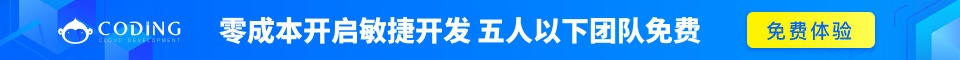# ASP.NET Razor VB 变量

## 变量

### 实例

// Using the Dim keyword:
Dim greeting = "Welcome to ziqiangxuetang.com"
Dim counter = 103
Dim today = DateTime.Today

// Using data types:
Dim greeting As String = "Welcome to ziqiangxuetang.com"
Dim counter As Integer = 103
Dim today As DateTime = DateTime.Today

## 数据类型

integer整数（全数字）103, 12, 5168
double64 位浮点数3.14, 3.4e38
decimal十进制数字（高精度）1037.196543
boolean布尔值true, false
string字符串"Hello ziqiangxuetang.com", "John"

## 运算符

VB 语言支持多种运算符。下面列出了常用的运算符：

=给一个变量赋值。i=6
+
-
*
/

i=5+5
i=5-5
i=5*5
i=5/5
+=
-=

i += 1
i -= 1
=相等。如果值相等则返回 true。if i=10
<>不等。如果值不等则返回 true。if <>10
<
>
<=
>=

if i<10
if i>10
if i<=10
if i>=10
&连接字符串（一系列互相关联的事物）。"w3" & "schools"
.点号。分隔对象和方法。DateTime.Hour
()圆括号。将值进行分组。(i+5)
()圆括号。访问数组或者集合的值。name(3)
And
OR

AndAlso
orElse

## 转换数据类型

AsInt()
IsInt()

myInt=myString.AsInt()
end if
AsFloat()
IsFloat()

myFloat=myString.AsFloat()
end if
AsDecimal()
IsDecimal()

myDec=myString.AsDecimal()
end if
AsDateTime()
IsDateTime()

myDate=myString.AsDateTime()
AsBool()
IsBool()

myBool=myString.AsBool()
ToString()转换任何数据类型为字符串。myInt=1234
myString=myInt.ToString()